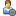###Show Posts

This section allows you to view all posts made by this member. Note that you can only see posts made in areas you currently have access to.

### Topics - Groit

Pages: 
1
##### Flat Earth Debate / Flaw in EP - detrimental to FET
« on: July 22, 2020, 04:12:21 PM »
I can see why the FES like to use Einstein's 'Equivalence Principle' to back up their theory especially where UA in concerned, and its true that you cannot distinguish between a uniformly accelerating frame and gravity when the accelerations are both 1g. Here is a few quotes from the FE wiki:

Quote
Inertial mass and gravitational mass are equivalent and it follows that kinematic acceleration and acceleration due to gravity are equivalent and indistinguishable from one another. &quot;Gravity&quot; is simply geometry.

Quote
Equivalence
To fully understand the Equivalence Principle, imagine you are in what looks to be the inside of an elevator. There are no windows and no way to see out of the container you are in. If you drop an object, say an apple for instance, and it falls to the floor in exactly the same manner in which you would expect it to on Earth. How can you tell whether the container you are in is being influenced by a massive object's gravitational field or if the container is being accelerated? The answer is, according to Einstein, is that you cannot. Let us now say that you start to feel a sense of weightlessness and begin to float around inside your container. How can you tell whether the container you are in is in deep space far from anything exhibiting a gravitational field or if you are free-falling with the container due to gravitation. Once again, you cannot.

Quote
Upward Acceleration
In terms of a Flat Earth, the equivalence principle asserts that acceleration and gravitation are identical and indistinguishable from one another. An object being accelerated at 9.81 m/s^2 will feel all the same effects as if it were in a gravitational field in which acceleration due to gravity is 1g.

All this is perfectly true for objects with mass such as humans falling towards the Earth, or tennis balls being tossed around, projectile motions etc... However, I cannot seem to find anything in the Wiki (maybe i missed it) on the way we observe light in both accelerating frames and gravity. The EP also states that observers in both accelerating frame and a gravitational field will detect the same Doppler effects in light, and depending on which way the photons are sent, we will observe a redshift or blueshift by the same amount. see below:This equivalence in Doppler shifts is only true when the accelerating rocket starts its acceleration. What I'm trying to say is that in gravity, like at the surface of the Earth, the observed Doppler shift is always the same and does NOT change with time. This was demonstrated in the Pound-Rebka experiment back in the 1960's where photons were dropped from a height of 22.6 m towards the surface of the Earth and they detected a blueshift (z) ofand the formula is given by:However, for an accelerating frame such as the rocket, the formula for the expected blueshift (z) is given by:and as you can see this is time dependant, whenin the denominator increases then the blueshift (z) becomes lower, there's a blueshift drift as time goes by. Here is a diagram and a link to the paper for redshift / blueshift drift for 'uniform accelerating frames':https://arxiv.org/pdf/1907.06332.pdf

Here's a plot showing how the blueshift drift against time for gravity and the accelerating frame such as a rocket or Universal Acceleration (Earth accelerating upwards) if the Pound-Rebka experiment was carried out in both cases. The time axis is for six months.Since the Pound-Rebka experiment did NOT detect any blueshift drift over time, then we can conclude that the results are experimental evidence that we are 100% in a gravitational field, and we are NOT accelerating as UA suggests. So if gravity exist then the Earth cannot be flat and that it must be round, and all objects on the surface are accelerating towards the centre of the round Earth due to gravity which is the curvature of spacetime.

Pages: